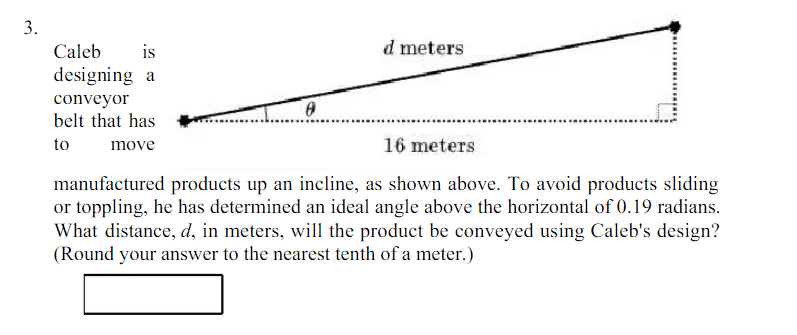# Right Triangle Problem

• MHB
• Jacob123

#### Jacob123

How would I have to calculate this question for an answer, a friend of mine told me he could get the answer without knowing that the bottom line was 16 meters, I can't seem to find a way that would work, I am not sure if I am missing something or he is lying.Last edited by a moderator:

I'd also like to know how your friend could determine a numerical value for $d$ just knowing the angle $\theta = 0.19$ with no other information.
Using the angle, $\theta$, value and the 16 meters ...
$\cos{\theta} = \dfrac{16}{d} \implies d = \dfrac{16}{\cos{\theta}}$